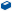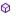Press on the image to return to the main documentation page.

# Astro

Astro

### Astro

The library provides data about the Sun and the Moon
like the times of Sunrise, Sunset, Moon rise and Moon set,
and more, according to place and date.
for the phase of the moon there are two algorithms, one is based on the
day of the moon month in the Hebrew Calendar, the other on the Julian calendar.
The Sunrise and Sunset times vary according to the value of Zenith angle.
The following conventions are in use:
Official 90.8333 (90� 50')
Civil - 96�
Nautical - 102�
Astronomical - 108�
The values can be used by their names to be selected
using the method Zenith.
The library uses the Official value as default,

The rise an set results were checked against this site
Sun & Moon Tables
and found accurate within one minute difference.

None

#### Members:ASTRONOMICAL As DoubleCIVIL As DoubleGetMoonData (day As Int, month As Int, year As Int, hour As Int, minute As Int, deltahour As Double) As Double()GetMoonData2 (julianday As Double) As Double()GetMoonDistance (day As Int, month As Int, year As Int, hour As Int, minute As Int, deltahour As Double) As DoubleGetMoonDistance2 (julianday As Double) As DoubleGetMoonLat (day As Int, month As Int, year As Int, hour As Int, minute As Int, deltahour As Double) As DoubleGetMoonLat2 (julianday As Double) As DoubleGetMoonLong (day As Int, month As Int, year As Int, hour As Int, minute As Int, deltahour As Double) As DoubleGetMoonLong2 (julianday As Double) As DoubleGetSunData (day As Int, month As Int, year As Int, hour As Int, minute As Int, deltahour As Double) As Double()GetSunData2 (julianday As Double) As Double()GetSunLong (day As Int, month As Int, year As Int, hour As Int, minute As Int, deltahour As Double) As DoubleGetSunLong2 (julianday As Double) As DoubleHebMoonPhase (day As Int, month As Int, year As Int) As IntJulianday (day As Int, month As Int, year As Int, hour As Int, minute As Int, deltahour As Double) As DoubleJulianday2 (datetime As Long, deltahour As Double) As DoubleMoon (day As Int, month As Int, year As Int, latitude As Double, longitude As Double, deltahour As Double) As String()NAUTICAL As DoubleOFFICIAL As DoubleSun (day As Int, month As Int, year As Int, latitude As Double, longitude As Double, deltahour As Double) As String()Zenith As Double

#### Members description:

#####GetMoonData (dayAsInt, monthAsInt, yearAsInt, hourAsInt, minuteAsInt, deltahourAsDouble) AsDouble()
Returns an array of 5 doubles:
- Moon's Age in days
- Moon's angular diameter in arcminutes
- Moon's brightness
#####GetMoonData2 (juliandayAsDouble) AsDouble()
Returns an array of 5 doubles:
- Moon's Age in days
- Moon's angular diameter in arcminutes
- Moon's brightness
using Julian Day as parameter.
#####GetMoonDistance (dayAsInt, monthAsInt, yearAsInt, hourAsInt, minuteAsInt, deltahourAsDouble) AsDouble
Returns the Moon's distance in Km.
#####GetMoonDistance2 (juliandayAsDouble) AsDouble
Returns the Moon's distance in Km, using Julian day as parameter.
#####GetMoonLat (dayAsInt, monthAsInt, yearAsInt, hourAsInt, minuteAsInt, deltahourAsDouble) AsDouble
Returns the Moon's Latitude.
#####GetMoonLat2 (juliandayAsDouble) AsDouble
Returns the Moon's Latitude, using Julian day as parameter.
#####GetMoonLong (dayAsInt, monthAsInt, yearAsInt, hourAsInt, minuteAsInt, deltahourAsDouble) AsDouble
Returns the Moon's Ecliptical Longitude.
#####GetMoonLong2 (juliandayAsDouble) AsDouble
Returns the Moon's Ecliptical Longitude, using Julian day as parameter.
#####GetSunData (dayAsInt, monthAsInt, yearAsInt, hourAsInt, minuteAsInt, deltahourAsDouble) AsDouble()
Returns an array of 3 doubles:
- Sun's distance in Astronomical Units AU
(1 Astronomical Unit = 149,598,000 kilometers)
- The Sun's angular diameter
- The sun's brightness (magnitude)
#####GetSunData2 (juliandayAsDouble) AsDouble()
Returns an array of 3 doubles:
- Sun's distance in Astronomical Units AU.
(1 Astronomical Unit = 149,598,000 kilometers)
- The Sun's angular diameter
- The sun's brightness (magnitude)
using Julian Day as parameter.
#####GetSunLong (dayAsInt, monthAsInt, yearAsInt, hourAsInt, minuteAsInt, deltahourAsDouble) AsDouble
Returns the ECLIPTICAL LONGITUDE of the sun.
#####GetSunLong2 (juliandayAsDouble) AsDouble
Returns the ECLIPTICAL LONGITUDE of the sun using Julian Day as parameter.
#####HebMoonPhase (dayAsInt, monthAsInt, yearAsInt) AsInt
Returns the phase of the moon as per the Hebrew calendar moon month.
#####Julianday (dayAsInt, monthAsInt, yearAsInt, hourAsInt, minuteAsInt, deltahourAsDouble) AsDouble
Returns the Julian Day.
deltahour is the local time offset.
#####Julianday2 (datetimeAsLong, deltahourAsDouble) AsDouble
Returns the Julian Day using DateTime parameter.
datetime is the local time in DateTime ticks.
deltahour is the local time offset.
#####Moon (dayAsInt, monthAsInt, yearAsInt, latitudeAsDouble, longitudeAsDouble, deltahourAsDouble) AsString()
Returns the Moonrise and Moonset times in an array of two strings.
If there is no rise or set at the specified date,
the returned string is "--:--".
#####Sun (dayAsInt, monthAsInt, yearAsInt, latitudeAsDouble, longitudeAsDouble, deltahourAsDouble) AsString()
Returns the Sunrise and Sunset times in an array of two strings.
At Latitudes greater then 65 or smaller then -65 there are cases that
there is no sunrise and sunset. The result in this case is "--:--"
#####ZenithAsDouble
Gets and sets the value of zenith.
Top